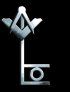Thu 30 Nov 2023 | Signed editions Find out more....

 Introduction The foreword toTurning The Solomon Keyby Katherine Neville Listen to Robert read fromTurning The Solomon Key Why Research Astrology? A Theory of Dowsing How Radio Works Newton's Rules of Logic Calculation of Ionicspheric Turbulence The Solomon Key Symbol Order your copy now! Websites of Interest Related books

## Calculation of Ionospheric Turbulence

### Robert Lomas

If I work out what happens to a single electron then I can estimate the total currents by substituting my calculation of the movement vector into the current density equation, derived from critical frequency observations.

I have imagined my model showing two gravitational attractors revolving round the Earth and pulling my representative floating electron in different directions as they traverse the sky.

My electron will be subject to similar forces to those which move the sea in and out with the pattern of the tides, but tides are damped movements because of the viscosity of the liquid in the sea. My representative electron was free to move in any direction as quickly or as slowly as it wanted, without any friction of water flow to slow it down. (Although if I come to refine the model I will need to take account of such effect as the Colomb back-scattering, caused by the electrons repelling each other if they get too close. But for the sake of this order of magnitude calculation I will ignore that complication.)

Here is a diagram of the model I used. The three small arrows show the direction of the gravitational forces on the electron.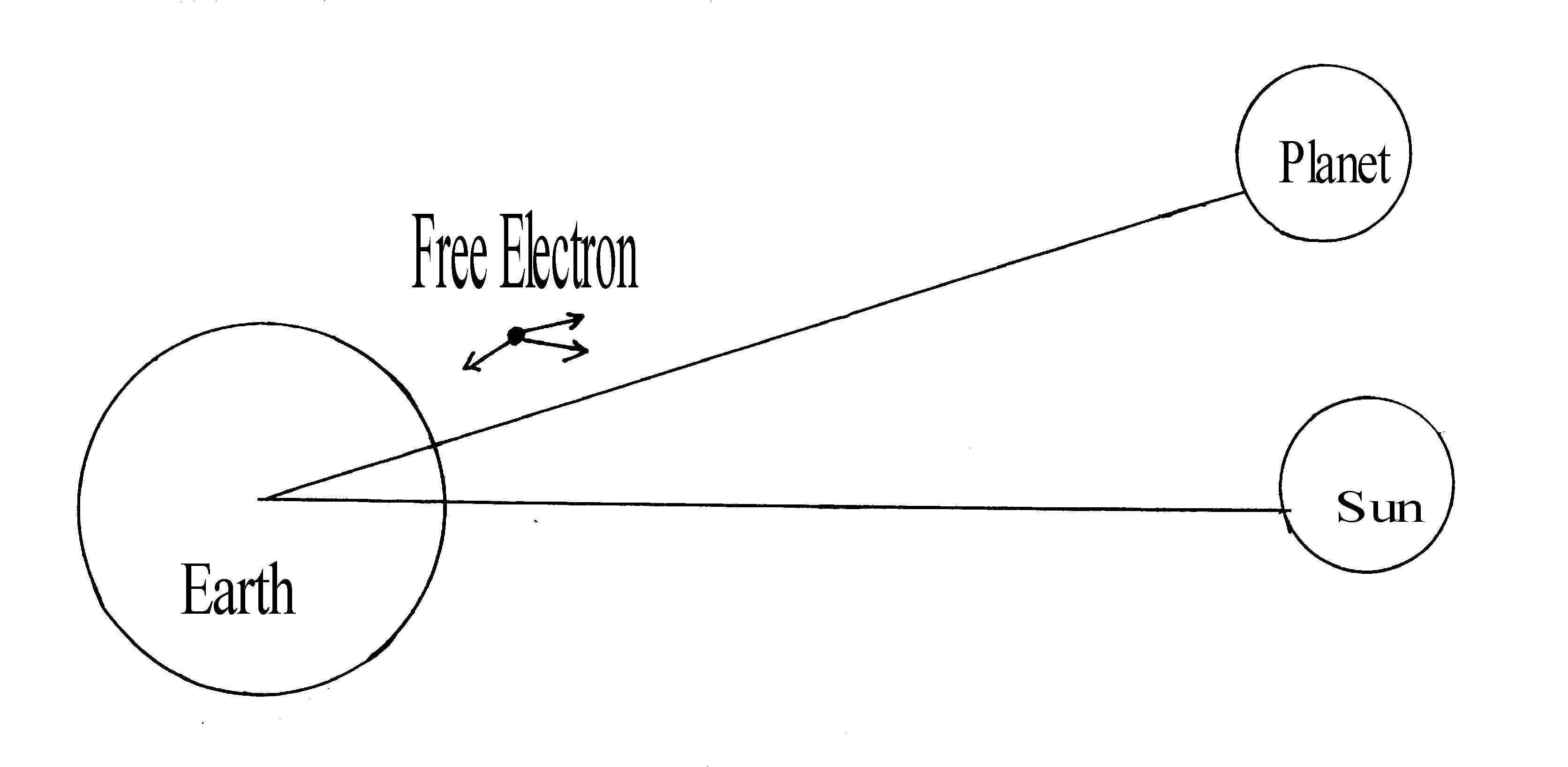To work out how the electron moves I need to work out the strength and direction of each of those forces. To do this I simply apply Newton's Law of Gravitational Attraction. The detail of this calculation it is shown below.

Now these three forces pulling at this individual free electron are not static, both the gravitational pull of the Sun and of the planet are rotating about the centre of mass of the Earth, which for ease of calculation I defined as my fixed reference point. The little electron is in the middle of a three way triangle of gravitational attractors all trying to pull it towards them and two of them moving rapidly across the sky, in a complex dance which some times brings them together but often moves them apart.

### Calculating the Movement of a Free Electron subject to Three Moving Gravitational Fields.

First I have to define some variables to use. Here they are:

Let me = the mass of the Earth

Let mp = the mass of the planet

Let ms = the mass of the Sun

Let m0 = the mass of the free electron

Let d1 = the distance between the free electron and the planet

Let d2 = the distance between the free electron and the Sun

Let h = the height of the ionised layer which contains the free electron

Let f1 = the gravitational force of the Earth on the electron

Let f2 = the gravitational force of the Sun on the electron

Let f3 = the gravitational force of the planet on the electron

Let G = Newton's general gravitational constant

The first step is to calculate these three forces in terms of the known parameters.

First the gravitational pull of the Earth on the electron: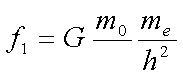Next the pull of the Sun on the same electron.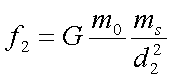Finally the pull of the planet on that poor much tugged electron.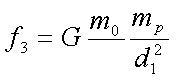If I am to discover anything about how these forces tug at the electron I must know how they main players are moving and to do that I have to have some line to measure from. I decided to define a line between the centres of mass of the Sun and the Earth at midnight GMT as my reference line. The angle that the Sun makes with this line is represented by Theta. The angle the planet makes with this same reference line is Phi. Both these angles change continuously but both the angles and their rates of change can easily be measured by standard astronomical techniques.

Using these time varying angles and their relationship to the previously calculated gravitational forces I can now work out the cumulative force on the electron along the line between the centres of mass of the Sun and the Earth (fv)and also at right angles to this line(fh), which is, the force parallel to the surface of the Earth. These two forces turned out to be a very interesting function of the Theta and Phi, as I have shown below.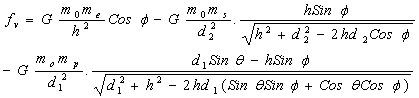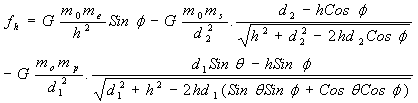The combination of these perpendicular and vertical forces is found by adding the two vectors thus to find the resultant or combined forces which I've called fr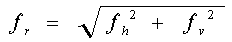At first sight this function looks a little complicated but it has some very clear features which indicate that it is going to cause turbulent behaviour. The interaction between the Sin and Cos functions is going to be very unstable around the points when either angle approaches 0, 90, 180, or 270 degrees. At this point one of the functions is rapidly approaching 1 whilst the other is approaching 0. When the number one is divided by zero, the function tries to become infinitely large. This means that when the gravitation fields of the Sun and planet occupy approximately the same angle in the sky; are opposite each other; or when they are at right angles to each other, the criss-crossing forces are really going to churn up the electron cloud and seriously rattle individual electrons about.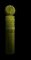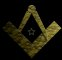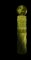Website by Glug. All content including images is copyright Robert Lomas, 2006-2023.